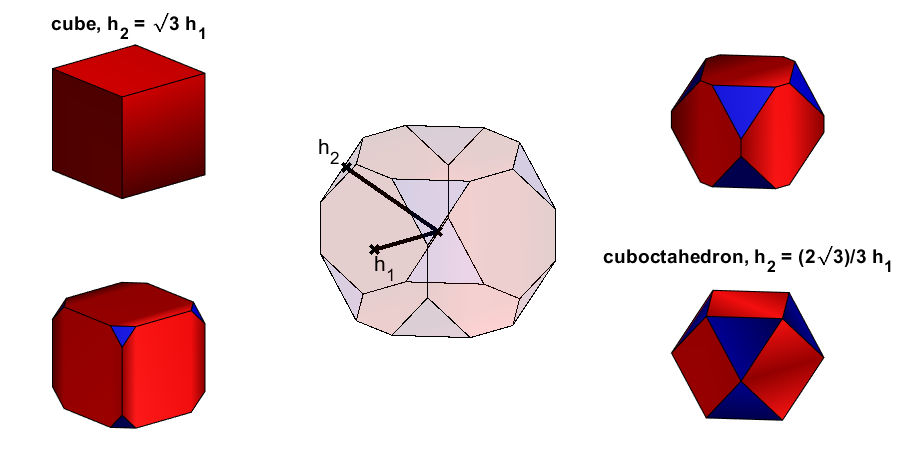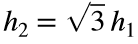# Problem 46746. Volume of a truncated cube

The surface of a truncated cube consists of 6 octagons, and 8 equilateral triangles. Our truncated cube is parametrised by (h1, h2), where
• h1 refers to the distance between the center of the volume and the center of the octagons, and
• h2 refers to the distance between the center of the volume and the center of the triangles.Forwe get the special case of a cube, and forwe get a cuboctahedron.
Your task: Write a function which returns the volume V of the truncated cube as function of the heights h1 and h2. If the ratio h2/h1 violates the constraintsreturn the volume of the related special case (cube or cuboctahedron) instead.

### Solution Stats

65.0% Correct | 35.0% Incorrect
Last Solution submitted on Nov 12, 2022

### Community Treasure Hunt

Find the treasures in MATLAB Central and discover how the community can help you!

Start Hunting!